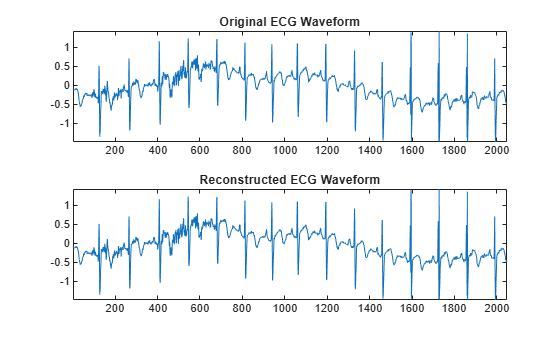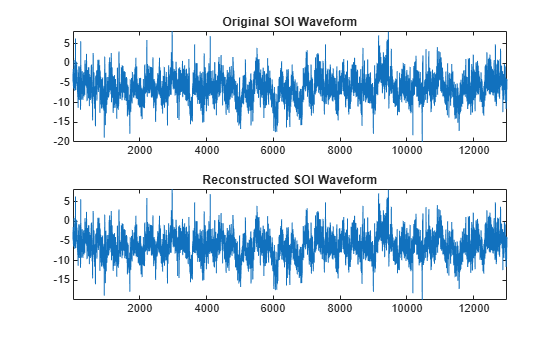Documentation

# imodwpt

Inverse maximal overlap discrete wavelet packet transform

## Syntax

``xrec = imodwpt(coefs)``
``xrec = imodwpt(coefs,wname)``
``xrec = imodwpt(coefs,lo,hi)``

## Description

example

````xrec = imodwpt(coefs)` returns the inverse maximal overlap discrete wavelet packet transform (inverse MODWPT), in `xrec`. The inverse transform is for the terminal node coefficient matrix (`coefs`) obtained using `modwpt` with the default length 18 Fejér-Korovkin (`'fk18'`) wavelet.```

example

````xrec = imodwpt(coefs,wname)` returns the inverse MODWPT using the orthogonal filter specified by `wname`. This filter must be the same filter used in `modwpt`.```

example

````xrec = imodwpt(coefs,lo,hi)` returns the inverse MODWPT using the orthogonal scaling filter, `lo`, and wavelet filter, `hi`.```

## Examples

collapse all

Obtain the MODWPT of an ECG waveform and demonstrate perfect reconstruction using the inverse MODWPT.

```load wecg; wpt = modwpt(wecg); xrec = imodwpt(wpt); subplot(2,1,1) plot(wecg); title('Original ECG Waveform'); subplot(2,1,2) plot(xrec); title('Reconstructed ECG Waveform');```Find the largest absolute difference between the original signal and the reconstruction. The difference is on the order of $1{0}^{-11}$, which demonstrates perfect reconstruction.

`max(abs(wecg-xrec'))`
```ans = 1.7903e-11 ```

Obtain the MODWPT of Southern Oscillation Index data using the Daubechies extremal phase wavelet with two vanishing moments (`'db2'`). Reconstruct the signal using the inverse MODWPT.

```load soi; wsoi = modwpt(soi,'db2'); xrec = imodwpt(wsoi,'db2');```

Obtain the MODWPT of Southern Oscillation Index data using specified scaling and wavelets filters with the Daubechies extremal phase wavelet with two vanishing moments (`'db2'`).

```load soi; [lo,hi] = wfilters('db2'); wpt = modwpt(soi,lo,hi); xrec = imodwpt(wpt,lo,hi);```

Plot the original SOI waveform and the reconstructed waveform.

```subplot(2,1,1) plot(soi) title('Original SOI Waveform'); subplot(2,1,2) plot(xrec) title('Reconstructed SOI Waveform')```## Input Arguments

collapse all

Terminal node coefficients of a wavelet packet tree, specified as a matrix. You must obtain the coefficient matrix from `modwpt` using the `'FullTree',false` option. `'FullTree',false` is the default value of `modwpt`.

Data Types: `double`

Synthesizing wavelet filter used to invert the MODWPT, specified as a character vector or string scalar. The specified wavelet must be the same wavelet as used in the analysis with `modwpt`.

Scaling filter, specified as an even-length real-valued vector. `lo` must be the same scaling filter as used in the analysis with `modwpt`. You cannot specify both a scaling-wavelet filter pair and a `wname` filter.

Wavelet filter, specified as an even-length real-valued vector. `hi` must be the same wavelet filter used in the analysis with `modwpt`. You cannot specify both a scaling-wavelet filter pair and a `wname` filter.

## Output Arguments

collapse all

Inverse maximal overlap discrete wavelet packet transform, returned as a row vector. The inverse transform is the reconstructed version of the original signal based on the MODWPT terminal node coefficients. `xrec` has the same number of columns as the input `coefs` matrix.

 Percival, D. B., and A. T. Walden. Wavelet Methods for Time Series Analysis. Cambridge, UK: Cambridge University Press, 2000.

 Walden, A.T., and A. Contreras Cristan. “The phase-corrected undecimated discrete wavelet packet transform and its application to interpreting the timing of events.” Proceedings of the Royal Society of London A. Vol. 454, Issue 1976, 1998, pp. 2243-2266.

##### Support평가판 신청